• Save

# Clock Problems with Solution and Answer for Competitive Exams

Last year 4.3K Views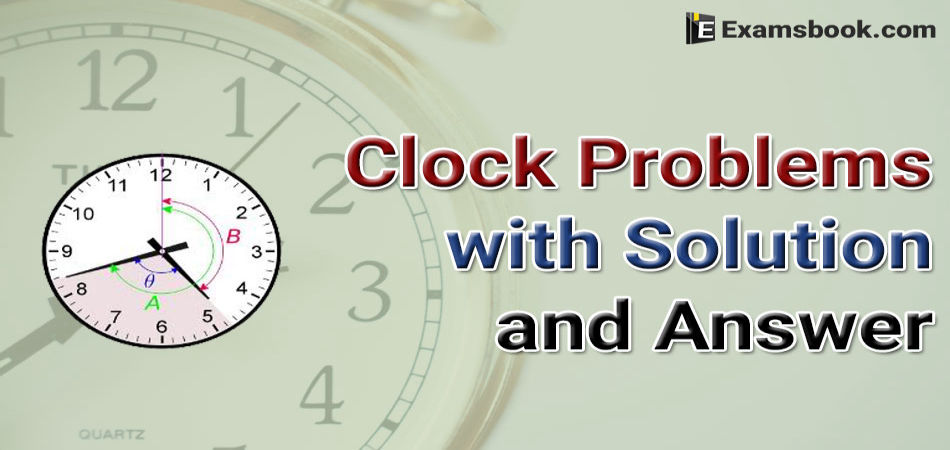Every student wants to get good marks in the competitive exams. For that students have to better their performance. To make a better performance, here I am sharing with you Clock Problems with solution and answer. These clock problems help you to solve clock based questions accurately.

So, keep practice with these clock problems with solutions and understand Clock Reasoning Formula with Examples to obtain a good score in the competitive exams.

## Clock Problems with Solutions and Answers

Q.1. At what time between 7 and 8 o'clock will the hands of a clock be in the same straight line but, not together?

(A)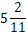min. past 7

(B)  5 min. past 7

(C)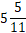min. past 7

(D)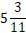min. past 7

Ans .  C

When the hands of the clock are in the same straight line but not together, they are 30 minute spaces apart.

At 7 o'clock, they are 25 min. spaces apart.

⸫ Minute hand will have to gain only 5 min. spaces.

55 min. spaces are gained in 60 min

5 min. spaces are gained in $$\left({60\over55}×5\right)min = 5{5\over 11}min$$

5 min. spaces are gained in

⸫ Required time =   $$\left(= 5{5\over 11}\right) min. past7$$

Q.2. An accurate clock shows 8 o'clock in the morning. Through how may degrees will the hour hand rotate when the clock shows 2 o'clock in the afternoon?

(A) 150°

(B) 144°

(C) 180°

(D) 168°

Ans .   C

Angle traced by the hour hand in 6 hours = $$=\left({360\over12}×6\right)^0=180^0$$

Q.3. At what time between 5.30 and 6 will the hands of a clock be at right angles?

(A)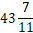min. past 5

(B)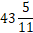min. past 5

(C) 45 min. past 5

(D) 40 min. past 5

Ans .  C

At 5 o'clock, the hands are 25 min. spaces apart.

To be at right angles and that too between 5.30 and 6, the minute hand has to gain (25 + 15) = 40 min. spaces.

55 min. spaces are gained in 60 min.

40 min. spaces are gained in $$\left({60\over55}×40\right)min = 43{7\over 11}min$$

∴   Required time = $$43{7\over 11}min. past 5$$

Q.4. The reflex angle between the hands of a clock at 10.25 is:

(A)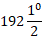(B) 180

(C)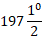(D) 195°

Ans .  C

Angle traced by hour hand in   $${125\over 12}hrs = \left({360\over12}×{125\over 12}\right)^0 = 312{1\over 2}^0$$

Angle traced by minute hand in 25 min $$= \left({360\over60}×25\right)^0 = 150^0$$

⸫ Reflex angle $$= 360^0 - \left(312{1\over 2}-150^0\right)=360^0-162{1^0\over 2}=197{1^0\over 2}$$

Q.5.The angle between the minute hand and the hour hand of a clock when the time is 4.20, is:

(A) 10°

(B) 0°

(C) 20°

(D) 5°

Ans .  A

Angle traced by hour hand in  $${13\over 3}hrs = \left({360\over 12}× {13\over 3} \right)^0 = 130^0$$

Angle traced by min. hand in 20 min =   $$\left({360\over 60}× 20 \right)^0 = 120^0$$

⸫ Required angle = (130 - 120)° = 10°

Q.6. A clock is started at noon. By 10 minutes past 5, the hour hand has turned through:

(A) 150°

(B) 145°

(C) 160°

(D) 155°

Ans .   D

Angle traced by hour hand in 12 hrs = 360°.

Angle traced by hour hand in 5 hrs 10 min. i.e.,   $$={31\over 6}\ hrs = \left({360\over 12}×{31\over 6}\right)=155^0$$

Q.7. At what angle the hands of a clock are inclined at 15 minutes past 5?

(A) 640

(B)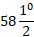(C)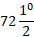(D)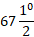Ans .  D

Angle traced by hour hand in $${21\over 4}= \left({360\over 12}×{21\over 4} \right)^0 = 157{1^0\over 2}$$

Angle traced by min. hand in 15 min. $$= \left({360\over 60}×15\right)^0=90^0$$

⸫ Required angle =   $$\left(157{1\over 2}\right)^0 - 90^0 = 67{1^0\over 2}$$

Q.8. A watch which gains 5 seconds in 3 minutes was set right at 7 a.m. In the afternoon of the same day, when the watch indicated quarter past 4 o'clock, the true time is:

(A) 4 p.m.

(B)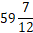min. past 3

(C)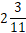min. past 4

(D)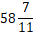min. past 3

Ans .  A

Time from 7 a.m. to 4.15 p.m. = 9 hrs 15 min. $$={37\over 4}hrs$$

3 min. 5 sec. of this clock = 3 min. of the correct clock.

⇒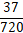hrs of this clock =hrs of the correct clock.

⇒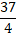hrs of this clock = $$\left({1\over 20}×{720\over 37}×{37\over 4} \right)hrs \ of \ the \ correct \ clock$$

= 9 hrs of the correct clock

⸫ The correct time is 9 hrs after 7 a.m. i.e., 4 p.m.

Q.9.At 3:40, the hour hand and the minute hand of a clock form an angle of:

(A) 125°

(B) 120°

(C) 135°

(D) 130°

Ans .   D

Angle traced by hour hand in 12 hrs. = 360°

Angle traced by it in $${11\over 3}hrs = \left({360\over 12}× {11\over 3} \right)^0 = 110^0$$

Angle traced by minute hand in 60 min. = 360°

Angle traced by it in 40 min =  $$\left({360\over 60}×40\right)^0 = 240^0$$

⸫ Required angle (240 - 110)° = 130°

Q.10. How much does a watch lose per day, if its hands coincide every 64 minutes?

(A)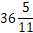min

(B)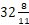min

(C) 96 min.

(D) 90 min.

Ans .   B

55 min. spaces are covered in 60 min.

60 min. spaces are covered in $$\left({60\over 50}×60\right)min = 65{5\over 11}min$$

Loss in 64 min. = $$=\left(65{5\over 11}-64\right)= {16\over 11} min$$

Loss in 24 hrs =   $$= \left({16\over11}×{1\over 64}×{24}×{60} \right)min = 32{8\over 11}min$$